# 5. Multiple Feature Representation Feedback

## 5. Multiple Feature Representation Feedback

An alternative to treating multiple multimedia features, as forming a single multidimensional space as discussed in section 4, is to treat each feature representation on its own. Each multimedia object o is represented by a collection of (multidimensional) feature values fij(o) each based on a different feature representation.

The query is represented using a two-level approach: a collection of individual feature queries and an aggregation function to combine the individual query distances into an overall distance.

The bottom level is formed by a collection C of single-feature representation queries qij where the query and feedback models are as described in section 4. When doing a search, the user can select which feature representation she is interested in including in the query, possibly even selecting different query and relevance feedback modes for different feature representations. Recall the example described in section 2 where there are two features: color and texture. For the color feature there are two representations: color histogram and color moments. For the texture feature, there are also two feature representations: wavelet and Tamura. As an example, a user can select for her query the color histogram feature representation using a single-point query model and query point movement for relevance feedback, and the wavelet feature representation using a multi-point based query model and multi-point query expansion for relevance feedback.  Given a multimedia object, the retrieval system computes the distance between the corresponding feature representation values in the multimedia object and each single feature representation query. In the above example, an object o can have a distance of 0.4 with respect to the color histogram query and a distance of 0.8 with respect to the wavelet texture query. Once the individual feature query distances have been computed for an object, they are aggregated together into an overall distance for that object. There are many ways to aggregate the distances with regard to individual feature representations; in this section we discuss an approach based on linear weighted summation. In this approach, we assign a weight wij to each single-feature representation query and use it to scale its distances. The overall distance is the sum of the scaled distances for all single-feature representation queries: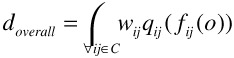and where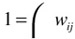. By qij (fij(o)) we mean the distance dijk based on the single-feature representation fij value from the multimedia object o, based on its single-feature query representation qij.  The user query for the above example becomes:

w1dcolor histogram (fcolor histogram (o), qcolor histogram) + w2dwavelet (fwavelet (o), qwavelet)

Suppose in our example w1=0.25 and w2=0.75 and the distance values shown above. The overall distance for object o is doverall=0.25*0.4 + 0.75*0.8= 0.1+0.6 = 0.7.

As in section 4, the retrieval system returns to the user a ranked list l=<a1, a2, > with the top results based on distance to the multi-feature query, and obtains relevance judgements rfs for the result objects as.

A query based on the multiple feature query model can change in two ways: by adding/deleting single-feature representation queries, and by changing the weights for each single-feature query called query re-weighting. First we discuss re-weighting based on the work in  and later how to add/delete from the query based on the work in .

### 5.1 Query Re-Weighting

Query re-weighting assumes that for the η independent single-feature representation queries qi, each with a corresponding weight wi, there exist a set of optimal weights wiopt that capture the user's information need. The initial query typically starts with default values for the wi's; typically they all start with the same value: wi=1/η.

To describe the re-weighting approach let us assume without loss of generality that there are only two single-feature queries: q1 and q2 and which return distances in the range [0,1]. Figure 21.5 shows a two dimensional space that shows the distances of q1 vs. q2. Since the overall distance function is a weighted summation of the component distances, we can represent the query as a line L. The distance of the line to the origin is determined by how many objects are returned; as more and more objects are returned to the user, the line moves away from the origin. The line Li represents the query at iteration i of relevance feedback, based on the weights w1i and w2i. Objects between this line and the origin have been returned and shown to the user, that is, the objects in the region OCB. Assuming that an optimal query with weights w1opt and w2opt exists, we can represent it with the line Lopt. Objects between this line and the origin are deemed relevant according to the user's information need, that is, objects in the region OAB are relevant. At this point, the user marks as relevant the points that she has seen and are relevant, that is, the points in the area OAEB. Assuming the points A and B are known, we can construct a new line Li+1 with weights w1i+1 and w2i+1 for iteration i+1 that crosses points A and B for a given set of returned objects. In  the authors show that the slope of Li+1 lies between the slopes of Li and Lopt. As the user goes through more relevance feedback iterations, the line Li+1 converges to the optimal line (weights) Lopt. What remains now is how to estimate the points A and B based on the results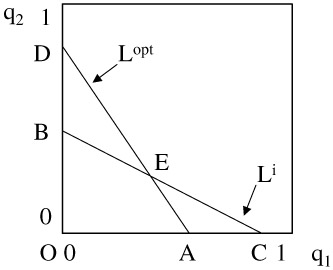Figure 21.5: Single-feature Distance Graph

shown to the user and her relevance feedback. We explore two strategies for deriving Li+1: (1) maximum distance and (2) counting.

Maximum distance strategy: Let rl be the set of objects marked relevant by the user (i.e., rf1). Let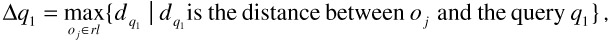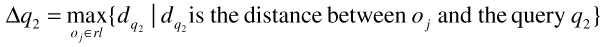We use Δq1 and Δq2 to estimate the points A and B in Figure 21.5. That is, point A corresponds to (Δq1,0) and point B corresponds to (0, Δq2). As a result, the slope of the line AB can be estimated as -Δq2/Δq1. Since the slope of the line Li+1 is the slope of the line AB, and given the constraint w1i+1+w2i+1 = 1, the weights are updated as follows: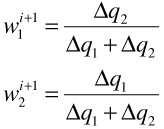Counting strategy: This approach attempts to measure the length of the lines OA and OB by counting the number of objects relevant to the user, which are close to the query based on individual feature queries q1 and q2. To see the motivation, consider Figure 21.6. All points between the origin O and the lines labelled cutq1 and cutq2 are very close to the query based on the single-feature queries q1 and q2 respectively. If we assume that objects are uniformly distributed in the similarity space of the user's query, the number of objects close to the query based on single-feature queries q1 and q2 that are also relevant to the overall query are proportional to the size of the regions OIGB and OAFH respectively. Since the lines cutq1 and cutq2 are introduced at equal distance thresholds, the size of the regions OAFH and OIGB is proportional to the lengths of the lines OA and OB. Let Λq1=cardinality of aj | dq1(aj)<cutq1, and Λq2=cardinality of aj | dq2(aj)<cutq2. As a result, the ratio Λq1/Λq2 gives the estimate of the slope of line DC. Given the estimate of the slope, and the constraint that w1i+1+w2i+1 = 1, a weight update policy is straightforward: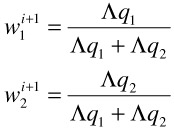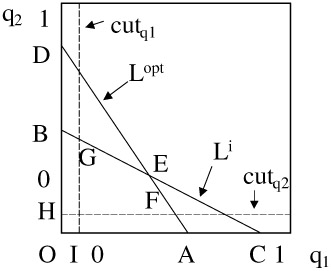Figure 21.6: Single-feature Distance Graph - Counting

A step beyond re-weighting is to add or delete single-feature representation queries to the overall query. Deletion occurs naturally when re-weighting causes a weight to fall to 0. This effectively nullifies any contribution by that feature and thus is eliminated from the overall query. Adding new single-feature queries is more interesting. We present the approach described in  which is more general than multimedia retrieval.

To find single-feature query candidates to add to the overall query, a set of candidate single-feature queries is formed. Each single-feature query is composed of a query point and distance function. The distance function is the default for the feature, and the query point is selected by choosing the corresponding feature representation value from the top ranked result aj with rfj>0, that is, the query value comes from the best relevant result. The candidate set can clearly be very large depending on the number of feature representations in the retrieval system. While restrictions can be placed on how many candidates to include (e.g., limited to those that can be evaluated in a given amount of time), in practice the number of feature representations is small enough to avoid serious problems. Using the candidate set, we construct a table that incorporates the final results <a1, a2, , am> shown to the user, their corresponding feedback values <rf1, rf2, , rfm>, and the distance between result aj and each single-feature candidate query ck. For example, if m=2 and there are two single-feature query candidates, the table is:

Result aj

Feedback rfj

c1 (aj)

c2 (aj)

a1

1 (relevant)

0.2

0.1

a2

0 (neutral)

0.5

0.7

We populate the column for each single-feature candidate query ck with the distances from ck to every result aj. For each column that represents a candidate query we compute the average and standard deviation between relevant and non-relevant results:Using this information, we compute the "separation" between relevant and non-relevant answers for each ck: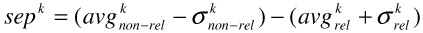We add ck to the overall query iff: sepk > 0 (v k) sepk > sepv, that is, we add ck to the query if there is at least one relevant and non-relevant standard deviation of separation between relevant and non-relevant values for ck, and choose the ck with the largest such separation. Experimental results in  show that this is a promising approach to adding component single-feature queries to the overall query.

### 5.3 Distance Normalization

In this discussion we have largely assumed that the distances between different feature representations are of comparable magnitudes. In general, this is not the case. For example, the distance values returned by one feature representation may lie in the range [0,1] while for another feature representation they may lie in the range [0,1000]. Weighting can help alleviate this disparity but is preferably not used for this purpose. It thus may become necessary to normalize the distance ranges between different feature representations and distance functions to make them of comparable magnitudes.

Among the many techniques to alleviate this problem are the min-max and Gaussian normalization approaches. In the min-max approach, the retrieval system keeps track of the minimum and maximum distances observed for each distance function and feature representation and normalizes the final distance by the distance range (maximum-minimum distance). The Gaussian approach advocated in , among others, proposes to treat all distances as a Gaussian sequence. To normalize distance values, they are divided by a multiple of the standard deviation to ensure most distances (99%) lie in the range [0,1]. The drawbacks of this technique are a high up-front normalization cost, and that it assumes a specific distribution of distance values which may not hold. In practice, as long as the distances for different feature representations and distance functions are normalized to a comparable range, few unwanted problems appear. Both approaches roughly perform equally well.

While this demonstrates the flexibility of this approach, in a realistic setting these choices may be hidden from the user and pre-arranged by the system developer.

Remember that this query representation may be any of those discussed in section 4.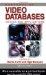Handbook of Video Databases: Design and Applications (Internet and Communications)
ISBN: 084937006X
EAN: 2147483647
Year: 2003
Pages: 393